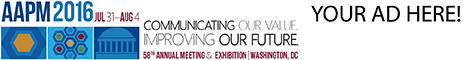# Program Information

## Analysis of the ArcCHECK Dosimetry Gamma Failure Using the 3DVH SystemS Cho

## Presentations

#### SU-E-T-117 (Sunday, July 12, 2015) 3:00 PM - 6:00 PM Room: Exhibit Hall

Purpose:To evaluate gamma analysis failure for the VMAT patient specific QA using ArcCHECK cylindrical phantom. The 3DVH system(Sun Nuclear, FL) was used to analyze the dose difference statistic between measured dose and treatment planning system calculated dose.

Methods:Four case of gamma analysis failure were selected retrospectively. Our institution gamma analysis indexes were absolute dose, 3%/3mm and 90%pass rate in the ArcCHECK dosimetry. The collapsed cone convolution superposition (CCCS) dose calculation algorithm for VMAT was used. Dose delivery was performed with Elekta Agility. The A1SL(standard imaging, WI) and cavity plug were used for point dose measurement. Delivery QA plans and images were used for 3DVH Reference data instead of patient plan and image. The measured data of ‘.txt’ file was used for comparison at diodes to acquire a global dose level. The *.acml’ file was used for AC-PDP and to calculated point dose.

Results:The global dose of 3DVH was calculated as 1.10 Gy , 1.13 , 1.01 and 0.2 Gy respectively. The global dose of 0.2 Gy case was induced by distance discrepancy. The TPS calculated point dose of was 2.33 Gy to 2.77 Gy and 3DVH calculated dose was 2.33 Gy to 2.68 Gy. The maximum dose differences were -2.83% and -3.1% for TPS vs. measured dose and TPS vs. 3DVH calculated respectively in the same case. The difference between measured and 3DVH was 0.1% in that case. The 3DVH gamma pass rate was 98% to 99.7%.

Conclusion:We found the TPS calculation error by 3DVH calculation using ArcCHECK measured dose. It seemed that our CCCS algorithm RTP system over estimated at the central region and underestimated scattering at the peripheral diode detector point. The relative gamma analysis and point dose measurement would be recommended for VMAT DQA in the gamma failure case of ArcCHECK dosimetry.

Contact Email: